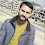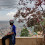### Introduction

When executing a particular task using algorithms, one common thing that hits every problem solver brain is the efficient and fast algorithm to solve that problem. But, what do exactly fast and efficient means? Is it the measure of real processing time? No, it's not the measure of real time like second and minutes because the old computer with Pentium processor may take a long time to process the same algorithm than the new Intel i3 processor, or, A bad algorithm written in Assembly may execute faster than a good algorithm written in python. So, run time of program can't be considered to measure algorithmic efficiency.

Hence, to measure the algorithmic efficiency, the concept of Asymptotic Complexity of a program and a notation for describing this called Big Oh  was introduced. Big Oh is the worst case, Big Omega and Big Theta are best and average case notations. Worst case means we are mostly unlucky for that problem domain , i.e precondition of task do not favour us. Complexity analysis is a tool that allows us to explain how an algorithm behaves as the input grows larger.

### Scenarios

Sorting an array of size 10000 compared to array of size 100 and analyzing how run time of the program grows.
Now, Lets dive deeper,
Counting the number of character in a string:

One simplest approach is traversing through whole string letter by letter and incrementing a counter variable by 1. This algorithmic approach run in linear time with respect to number of character 'n' in the string, i.e, it runs in O(n).
Why ??
Using this approach the time required to traverse the entire string is proportional to number of character in string, i.e time required to traverse string with 40 character is twice the time required to trverse string with 20 character as the amount of time to look individual character is same.

Another approach is, declaring a variable and storing the number of character in a variable say "length" early in the program, i.e, before storing the very first character. Now, there is no need to look at string, instead one have to check the value of that variable. The accessing of such variable is generally asymptotically constant time operation, or O(1). This is because Asymptotic means "How the run time change as input grows". In this approach the length of string whether it has one character or thousandsof character, the only thing we need to do is to find string length and which can be done by reading the "length" variable and the reading time for this variable is constant regardless of string size. Hence this approach can be referred as running in constant time.
The O(1) does not change with the size of inputs.

### Variations

There are many different Big Oh runtimes like O(n), O(n²) etc
O(n²) are asymptotically slower than O(n) i.e if n grows  O(n²) will take more time than O(n).
This dosen't means O(n) always run faster, maybe if input is smaller then  O(n²) may work faster yet unnoticed
Similarly, we may have logarithmic O(log(n)) for some cases like in Binary search. Binary search cut the array size in half with each operation.
Simpler Program can be anlysed by counting number of nested loop.
• A single loop over n items has O(n) complexity.
• A loop within a loop has O(n²) complexityBig Oh Variant

1.Nice article Shiva. One question though, is there any measure or mathematics to calculate the complexity in terms of Big Oh notation. Any empirical process at least might be helpful.

thanks

2.This comment has been removed by the author.

3.Good analysis and well elaborated. Good job man :)

1.Thank you Mr. Suresh

4.It is nice blog Thank you porovide important information and i am searching for same information to save my time Ruby on Rails Online Training Hyderabad

5.6.7.